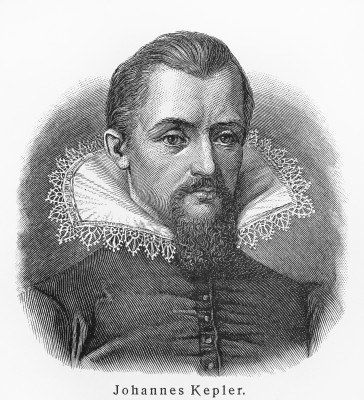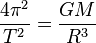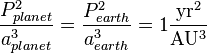The following are examples of formulas, laws, axioms, etc., that may be of benefit to the Kabalist. The purpose of studying the formulas is to establish certain principles within ourselves which represent or point us towards Universal Truths.

The real benefit of studying Esotericism is that it gives access to these Universal Truths, so that we can know why things happen the way they do, learn how to apply them in our lives, and then use them to accomplish our goals in the future.

In some of the formulas given, the capital Greek letter Sigma (Σ) is used, which (in math) represents a summation of many similar terms.

###Kepler’s Formulae or Laws

Kepler’s laws of planetary motion are three scientific laws describing orbital motion, originally formulated to describe the motion of planets around the Sun. Johannes Kepler published his first two laws in 1609, having found them by analyzing the astronomical observations of Tycho Brahe. Kepler discovered his third law many years later, and it was published in 1619. Kepler’s laws and his analysis of the observations on which they were based challenged the long-accepted geocentric models of the solar system, by asserting that the Earth orbited the Sun, proving that the planets’ speeds varied, and using elliptical orbits rather than circular orbits with epicycles.

1. First Law: The orbit of every planet is an ellipse with the Sun at one of the two foci.

Explanation: Symbolically, an ellipse can be represented in polar coordinates where (r, θ) are the polar coordinates (from the focus) for the ellipse, p is the semi-latus rectum (also called a conic section or just conic: which is a curve obtained as the intersection of a cone with a plane), and ε is the eccentricity of the ellipse. For a planet orbiting the Sun, r is the distance from the Sun to the planet and θ is the angle between the planet’s current position and its closest approach, with the Sun as the vertex.

 Formula:2. Second Law: A line joining a planet and the Sun sweeps out equal areas during equal intervals of time.

Explanation: Kepler’s 2nd Law is represented as the below formula where T is the period, G is Newton’s constant of gravity, M is the mass of the larger body, and R is the distance between the centers of mass of the two bodies.

 Formula:3. Third Law: The square of the orbital period of a planet is directly proportional to the cube of the semi-major axis of its orbit.

Explanation: The third law captures the relationship between the distance of planets from the Sun, and their orbital periods. The law can be expressed in the following formula where P is the orbital period of the planet and a is the semi-major axis of the orbit and for a sidereal year (yr), and astronomical unit (AU).

 Formula:### Newton’s Formulae or Laws

Newton’s laws of motion are three physical laws that form the basis for classical mechanics. The three laws of motion were first compiled by Sir Isaac Newton in his work Philosophiæ Naturalis Principia Mathematica [Latin for “Mathematical Principles of Natural Philosophy”] (1687). Newton used them to explain and investigate the motion of many physical objects and systems. For example, in the third volume of the text, Newton showed that these laws of motion, combined with his law of universal gravitation, explained Kepler’s laws of planetary motion.

1. First Law: Every body perseveres in its state of rest, or of uniform motion in a straight line, unless it is compelled to change that state by forces impressed upon it.

Explanation: If an object experiences no net force, then its velocity is constant: the object is either at rest (if its velocity is zero), or it moves in a straight line with constant speed (if its velocity is nonzero).

 Formula:2. Second Law: The alternation of motion is always proportional to the motive force impressed upon it; and is made in the direction of the straight line from which that force is impressed.

Explanation: The acceleration (a) of a body is parallel and directly proportional to the net force (F) acting on the body, is in the direction of the net force, and is inversely proportional to the mass (m) of the body, ie F = ma

 Formula:3. Third Law: For every Action there is always an opposed equal Reaction: or the mutual actions of two bodies upon each other are always equal, and direct to contrary parts.

Explanation: When a first body exerts a force (F1) on a second body, the second body simultaneously exerts a force (F2 = −F1) on the first body. This means that F1 and F2 are equal in magnitude and opposite in direction.

 Formula:Newton’s law of universal gravitation is a general physical law derived from empirical observations by what Newton called induction. It is a part of classical mechanics and was also formulated in Newton’s Philosophiæ. When Newton’s book was presented to the Royal Society in 1686, Robert Hooke made a claim that Newton had obtained the inverse square law from him.

• Law of Universal Gravitation: Every point mass in the universe attracts every other point mass with a force that is directly proportional to the product of their masses and inversely proportional to the square of the distance between them.

Explanation: Every point mass attracts every single other point mass by a force pointing along the line intersecting both points. The force is proportional to the product of the two masses and inversely proportional to the square of the distance between them. In the below formula F is the force between the masses, G is the gravitational constant, m1 is the first mass, m2 is the second mass, and r is the distance between the centers of the masses.

 Formula:###In 1811 Wronski published a paper entitled Introduction à la philosophie des mathématiques, et technie de l’algorithmie or ‘Introduction to the philosophy of mathematics, and [the] technique of the algorithm’, claiming that all modern mathematical formulas can be derived from his formula “Lex Suprema” or ‘Supreme Law’ of mathematics. As Wronski puts it: “In the absolute principle of mathematics, that is to say, in the PRIMITIVE IDENTITY of the primordial elements (of both the finite and the infinite), this Supreme Law offers its primordial elements to the law of creation, through which all other laws are derived.”

In Volume 1 of Wronski’s series Réforme Absolue du Savoir Humain or ‘Absolute Reform of Human Knowledge’ (1847), there are three laws given [on pages p.10, p.19 and p.25]. Wronski calls these laws the “Emblem of Messianism … the prototype for human knowledge” and says that they must “be used for a definitive reform of all the sciences, even of philosophy…”

• First fundamental law of mathematics: Lex Suprema or the Supreme Law

Explanation: The first law determines in a systematic and absolute manner the angular movement of the stars. In the formula below, Α (alpha) are determinants used to compute the coefficients (now known as Wronskians), and Ω (omega) are arbitrary functions, of which all the conceivable modes of the generation of quantities or of a function which is represented by Fx .

 Formula:• Second fundamental law of mathematics: Problema Universale or the Universal Problem

Explanation: The second law determines in a systematic and absolute manner, for all the angular positions of the stars, their distance from the central star. The Universal Problem serves to determine (for some order) the differentials of the function of a particular number who’s variables themselves are functions of another number who’s variables are independent. To solve this equation, what must be determined is the unknown quantity of x . In the below formula, the characteristics f, f1, f2, f3, etc., denote the functions of the unknown x, and the quantities x1, x2, x3, etc., are known or given.

 Formula:• Third fundamental law of mathematics: Teleiogis or the Law of Finality (Teleology is the philosophical doctrine that final cause, design, and purpose exist in nature)

Explanation: The third law determines the unity of measures of the periodicity of movements. Again the objective is to determine the value of x, which governs the CONGRUENCE or the CONCORDANCE between the three primitive elements of the algorithm: gradation, summation, and reproduction. In the formula and algorithm below, the gradation is xm (power), the summation is a (residue), the reproduction is M (module), and nombre entier is a whole number.

 Formula:Algorithm:Some Kabalists have suggested that the “Lex Suprema” shows many different concepts including that ‘infinity equals the sum of all possible qualities/quantities’.

While many in the mathematical community have been slow to accept Wronski’s ideas and may still consider his formulas flawed or not universally applicable, the idea that anything and everything can be synthesized into an algebraic equation or algorithmic formula is a beautiful concept which gives wonderful insight into the study of Kabalah.

### Eliphas Levi’s Formulae or Axioms

There are many Axioms given in all of Levi’s works, here we present only a few. The first is from the ‘Introduction’ to History of Magic, the second is from Ch. 20 of Dogma of High Magic, and the third is from a letter #34 (dated February 18th, 1862) to his student Baron Spédalieri:

• The Divine Balance rules and necessitates Eternal Mathematics
• We are the authors of our own destiny, and God does not save us without our help.
• To really merit or deserve something from God, one must obey God. But to obey God one must have an independent will. The slave does not obey, he undergoes. [Note that the slave ‘undergoing’ is similar to Gurdjieff’s concept of how things just ‘happen’ to mechanical humans because they cannot do ]

### Samael Aun Weor’s Formulae or Axioms

Returning now to The Path of Initiation (Imagination, Inspiration, Intuition) from Ch. 85 of Tarot and Kabalah, lets take a look at the interesting statements made that we could consider Axioms for understanding the Path of Initiation:

• No phenomenon of Nature can be integrally comprehended if we consider it isolated. Everything is in incessant movement, everything changes, nothing is still.
• Matter is Condensed Energy.
• An object is a point in space which serves as a vehicle to determined sums of values.
• Life is … determined and determining Energy. Life is Subject and Object at the same time.